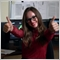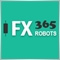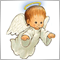# A question about modify StopLoss and TakeProfit order ...372

Hi all,

In my EA it will open a buy or sell order according to the conditon. If an order is opened, then I will add the SL and TP order by using the ModifyOrder as follow ( here is a buy order for example):

```...
extern double SL_prozent = 0.5;
extern double TP_prozent = 5;

...
// Handle Long Signal
if(bEntryLong){
if(iTotal>0)
closeAllOpenedShortOrders();
else{
if(iLongTicket>0)
{
Print("LONG order opened : ",OrderOpenPrice());
if(bStopLossAndTakeProfit){
if(OrderCloseTime() == 0 && OrderStopLoss() == 0)
{
//double StoppLoss = NormalizeDouble(OrderOpenPrice() / ( 1 + SL_prozent/100), Digits);
double StopLoss = NormalizePrice(OrderOpenPrice() / ( 1 + SL_prozent/100));
bool OrderAngepasst = OrderModify(OrderTicket(),OrderOpenPrice(),StopLoss,OrderTakeProfit(),0,Yellow);
if(!OrderAngepasst)
Print("Error by OrderModify : ",GetLastError());
else
Print("Order modified successfully.");
}

if(OrderCloseTime() == 0 && OrderTakeProfit() == 0)
{
//double TakeProfit = NormalizeDouble(OrderOpenPrice() * ( 1 + (TP_prozent /100)), Digits);
double TakeProfit = NormalizePrice(OrderOpenPrice() * ( 1 + TP_prozent/100));
bool OrderAngepasst = OrderModify(OrderTicket(),OrderOpenPrice(),OrderStopLoss(),TakeProfit,0,Orange);
if(!OrderAngepasst)
Print("Error by OrderModify : ",GetLastError());
else
Print("Order modified successfully.");
}

}
}
else
Print("Error by OrderSelect : ",GetLastError());
}
else
Print("Error opening LONG order : ",GetLastError());
}
}

...

double NormalizePrice(double price)
{
double tickSize = MarketInfo(Symbol(), MODE_TICKSIZE);
return( MathRound(price/tickSize) * tickSize );
} ```

There is no any problem by backtesting. But by paper trade or real trade, I notice only the TP order is set. The ST order not. Here is what I get from the "Experts":

```2018.05.08 17:00:00.232 EA_T05_MURI_Long_Short CFDDAX,M5: Order modified successfully.
2018.05.08 17:00:00.232 EA_T05_MURI_Long_Short CFDDAX,M5: modify #18175987 buy 0.20 CFDDAX at 12886.70 sl: 0.00 tp: 13531.04 ok
2018.05.08 17:00:00.170 EA_T05_MURI_Long_Short CFDDAX,M5: Order modified successfully.
2018.05.08 17:00:00.170 EA_T05_MURI_Long_Short CFDDAX,M5: modify #18175987 buy 0.20 CFDDAX at 12886.70 sl: 12822.59 tp: 0.00 ok
2018.05.08 17:00:00.106 EA_T05_MURI_Long_Short CFDDAX,M5: LONG order opened : 12886.7
2018.05.08 17:00:00.106 EA_T05_MURI_Long_Short CFDDAX,M5: Current Time: 2018.05.08 18:00:00 Ask: 12886.7 Bid: 12883.9 Spread: 2.800000000001091
2018.05.08 17:00:00.106 EA_T05_MURI_Long_Short CFDDAX,M5: open #18175987 buy 0.20 CFDDAX at 12886.70 ok
```

One can see the SL order was set successfully first:

```2018.05.08 17:00:00.170 EA_T05_MURI_Long_Short CFDDAX,M5: Order modified successfully.
2018.05.08 17:00:00.170 EA_T05_MURI_Long_Short CFDDAX,M5: modify #18175987 buy 0.20 CFDDAX at 12886.70 sl: 12822.59 tp: 0.00 ok```

And then the TP order was also set successfully. But the SL order was clean. At the end, only the TP order is set.

Where did I do wrong?48544

If you modify together SL and TP from the first time you may not have the same problem.5392

Hello Thomas, Use the below code for setting SL and TP:

```if(OrderCloseTime() == 0 && OrderStopLoss() == 0)
{
double StopLoss = NormalizePrice(OrderOpenPrice() / ( 1 + SL_prozent/100));
double TakeProfit = NormalizePrice(OrderOpenPrice() * ( 1 + TP_prozent/100));
bool OrderAngepasst = OrderModify(OrderTicket(),OrderOpenPrice(),StopLoss ,TakeProfit,0,Orange);
if(!OrderAngepasst)
Print("Error by OrderModify : ",GetLastError());
else
Print("Order modified successfully.");
}```3025

thomas2004:

Hi all,

In my EA it will open a buy or sell order according to the conditon. If an order is opened, then I will add the SL and TP order by using the ModifyOrder as follow ( here is a buy order for example):

There is no any problem by backtesting. But by paper trade or real trade, I notice only the TP order is set. The ST order not. Here is what I get from the "Experts":

One can see the SL order was set successfully first:

And then the TP order was also set successfully. But the SL order was clean. At the end, only the TP order is set.

Where did I do wrong?

If you have a reason to modify SL and TP separately (I don't think that is a good idea) you should call OrderSelect() again before modifying TP.372

Thanks Gyunay and Petr, I will have a try.372

Nedyalka Zhelyazkova:
If you modify together SL and TP from the first time you may not have the same problem.

This is not true. I've restarted my EA many times and everytime the same.372

Gyunay Sali:

Hello Thomas, Use the below code for setting SL and TP:

Hi Gyunay,

```if(OrderCloseTime() == 0 && OrderStopLoss() == 0 && OrderTakeProfit() == 0){
...
...
}

or

if(OrderCloseTime() == 0 && (OrderStopLoss() == 0 || OrderTakeProfit() == 0)){
...
...
}
```5392

Hi again,

It will be better to use this one:

```if(OrderCloseTime() == 0 && (OrderStopLoss() == 0 || OrderTakeProfit() == 0)){
...
...
}```
You will guarantee that even one of the SL or TP is 0 the code will run and you will set the missing value for SL or TP.372

Gyunay Sali:

Hi again,

It will be better to use this one:

You will guarantee that even one of the SL or TP is 0 the code will run and you will set the missing value for SL or TP.

Many thanks!5392

thomas2004:

Many thanks!

You are welcome :)